Question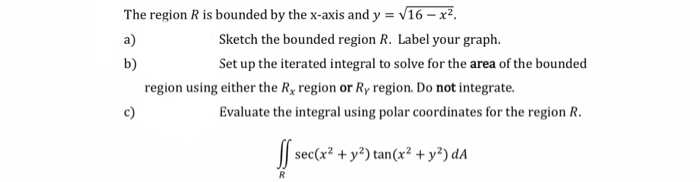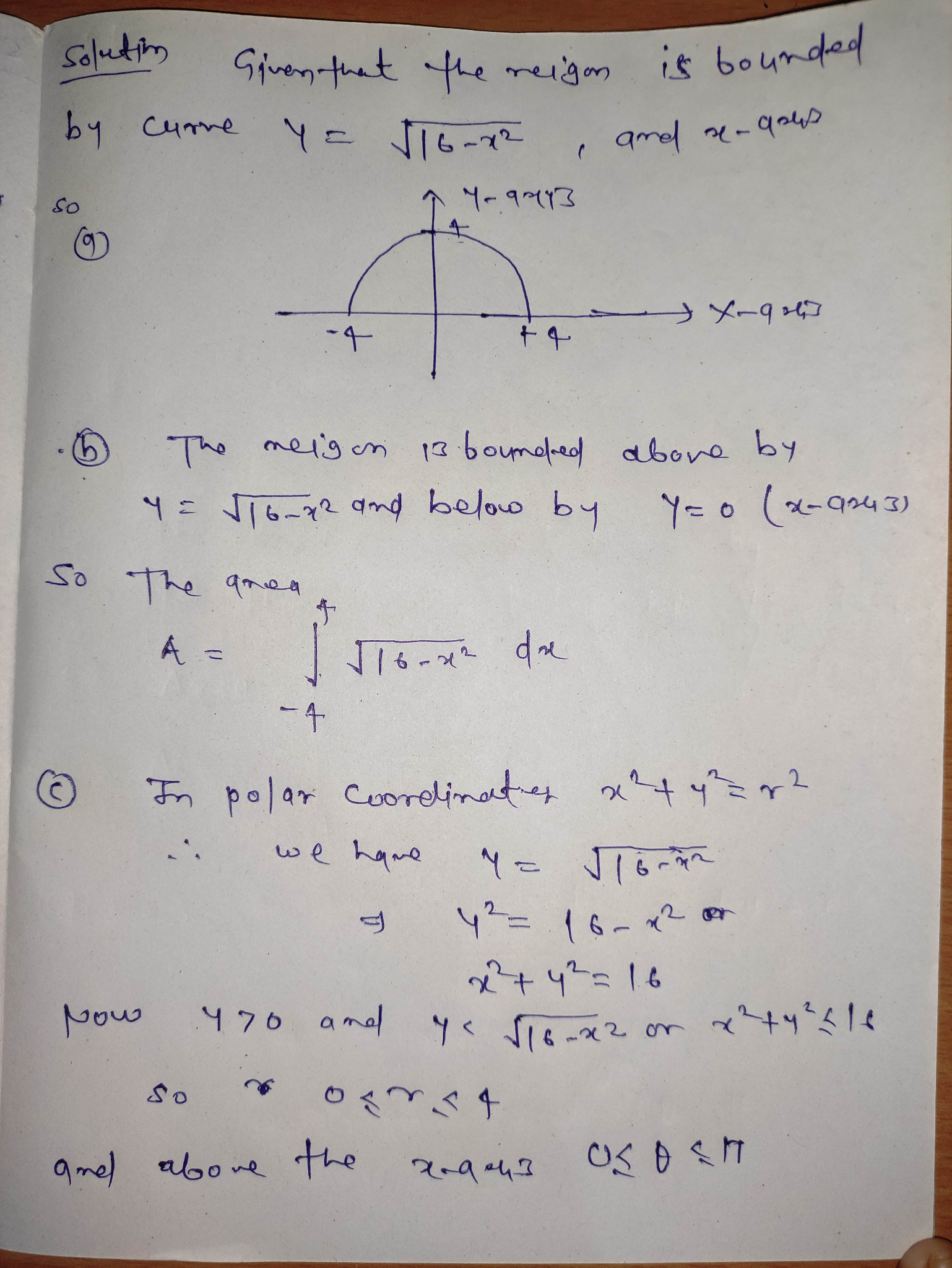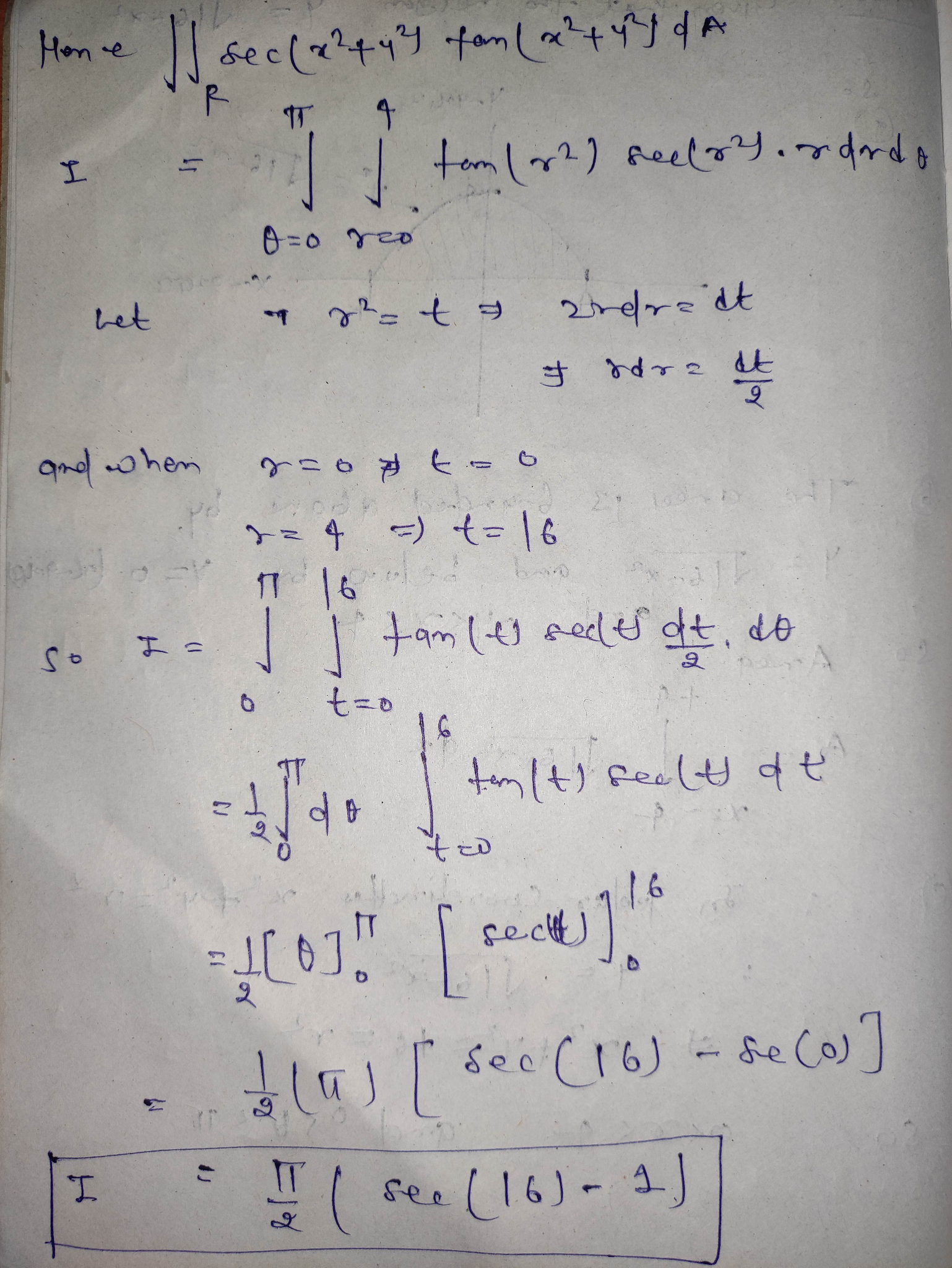#### Earn Coins

Coins can be redeemed for fabulous gifts.

Similar Homework Help Questions
• ### 2) The region R is bounded by the x-axis and y = V16 - x2 a)...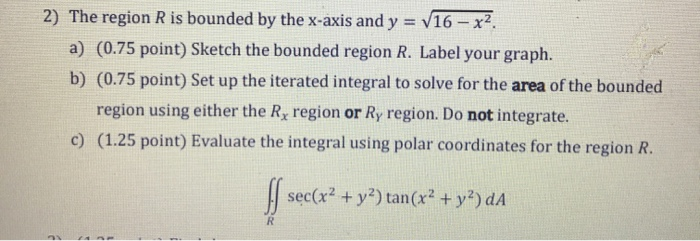2) The region R is bounded by the x-axis and y = V16 - x2 a) (0.75 point) Sketch the bounded region R. Label your graph. b) (0.75 point) Set up the iterated integral to solve for the area of the bounded region using either the Rx region or Ry region. Do not integrate. c) (1.25 point) Evaluate the integral using polar coordinates for the region R. sec(x2 + y2) tan(x2 + y2) dA R

• ### 2) The region R is bounded by the x-axis and y = V16 – x2. a)...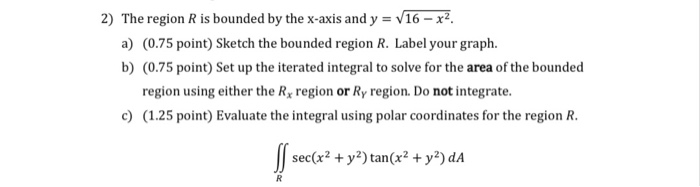2) The region R is bounded by the x-axis and y = V16 – x2. a) (0.75 point) Sketch the bounded region R. Label your graph. b) (0.75 point) Set up the iterated integral to solve for the area of the bounded region using either the Rx region or Ry region. Do not integrate. c) (1.25 point) Evaluate the integral using polar coordinates for the region R. S sec(x2 + y2) tan(x2 + y2) da R

• ### 2) The region R is bounded by the x-axis and y = V16 – x2 a)...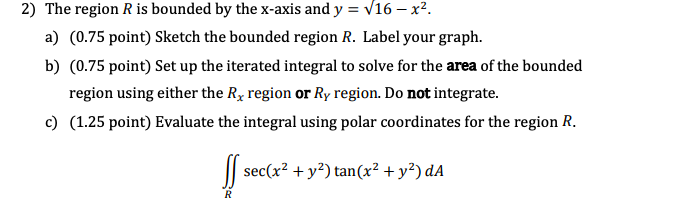2) The region R is bounded by the x-axis and y = V16 – x2 a) (0.75 point) Sketch the bounded region R. Label your graph. b) (0.75 point) Set up the iterated integral to solve for the area of the bounded region using either the Rx region or Ry region. Do not integrate. c) (1.25 point) Evaluate the integral using polar coordinates for the region R. S sec(x2 + y2) tan(x2 + y2) da R

• ### 2) The region R is bounded by the x-axis and y = V16 - x2 a)...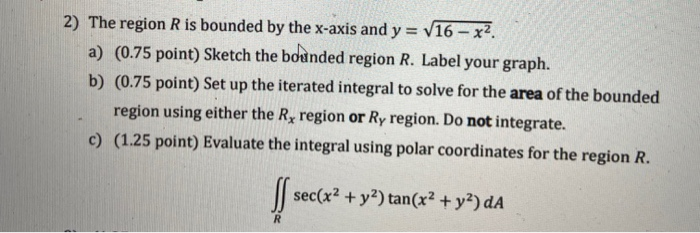2) The region R is bounded by the x-axis and y = V16 - x2 a) (0.75 point) Sketch the bounded region R. Label your graph. b) (0.75 point) Set up the iterated integral to solve for the area of the bounded region using either the Ry region or Ry region. Do not integrate. c) (1.25 point) Evaluate the integral using polar coordinates for the region R. sec(x2 + y2) tan(x2 + y2) dA R

• ### Show all of your work. Label the axes and scale on your graph. The sketch should...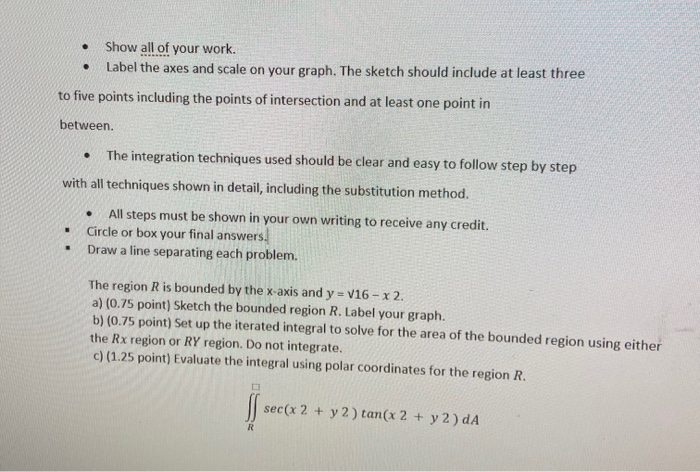Show all of your work. Label the axes and scale on your graph. The sketch should include at least three . to five points including the points of intersection and at least one point in between The integration techniques used should be clear and easy to follow step by step with all techniques shown in detail, including the substitution method. . . All steps must be shown in your own writing to receive any credit. Circle or box your final...

• ### 6. (4 pts) Consider the double integral∫R(x2+y)dA=∫10∫y−y(x2+y)dxdy+∫√21∫√2−y2−√2−y2(x2+y)dxdy.(a) Sketch the region of integration R in Figure 3.(b)...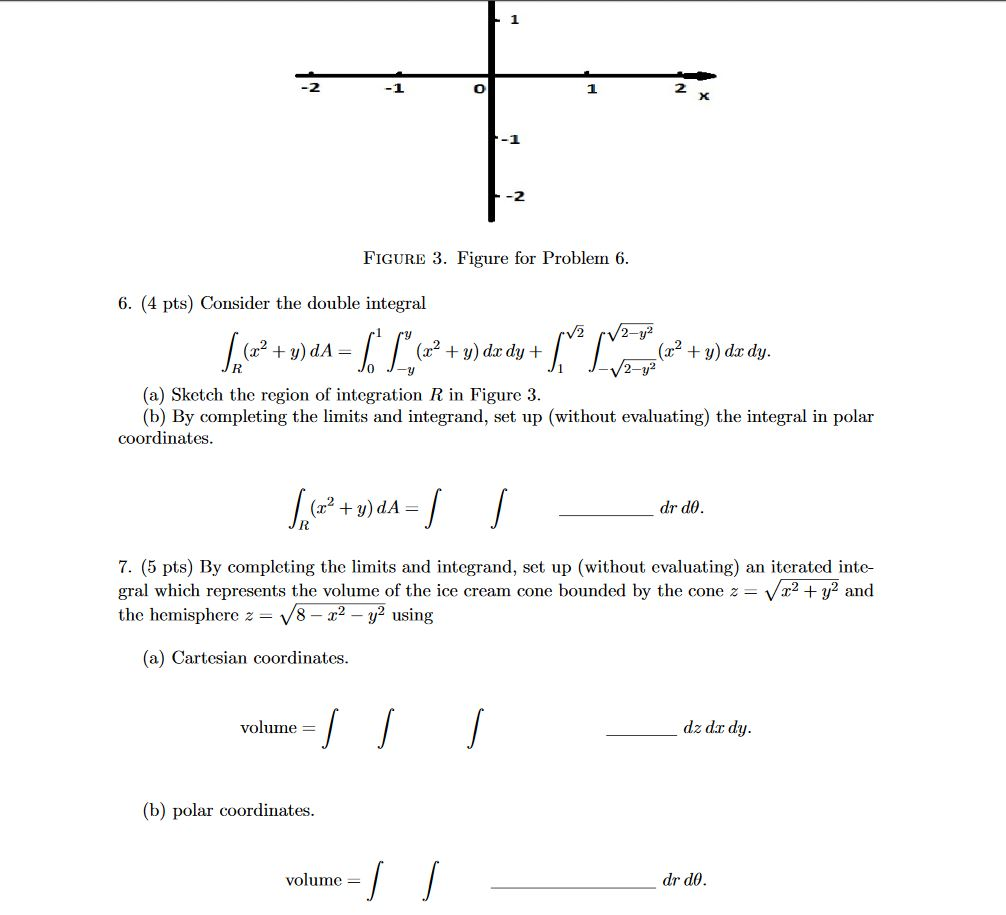6. (4 pts) Consider the double integral∫R(x2+y)dA=∫10∫y−y(x2+y)dxdy+∫√21∫√2−y2−√2−y2(x2+y)dxdy.(a) Sketch the region of integration R in Figure 3.(b) By completing the limits and integrand, set up (without evaluating) the integral in polar coordinates.∫R(x2+y)dA=∫∫drdθ.7. (5 pts) By completing the limits and integrand, set up (without evaluating) an iterated inte-gral which represents the volume of the ice cream cone bounded by the cone z=√x2+y2andthe hemisphere z=√8−x2−y2using(a) Cartesian coordinates.volume =∫∫∫dz dxdy.(b) polar coordinates.volume =∫∫drdθ. -1 -2 FIGURE 3. Figure for Problem 6. 6. (4 pts)...

• ### 6. (4 pts) Consider the double integral∫R(x2+y)dA=∫10∫y−y(x2+y)dxdy+∫√21∫√2−y2−√2−y2(x2+y)dxdy.(a) Sketch the region of integrationRin Figure 3.(b) By completing...6. (4 pts) Consider the double integral∫R(x2+y)dA=∫10∫y−y(x2+y)dxdy+∫√21∫√2−y2−√2−y2(x2+y)dxdy.(a) Sketch the region of integrationRin Figure 3.(b) By completing the limits and integrand, set up (without evaluating) the integral in polar coordinates. -1 -2 FIGURE 3. Figure for Problem 6. 6. (4 pts) Consider the double integral V2 /2-y² + = (x2 + y) dx dy + + y) do dy. 2-y2 (a) Sketch the region of integration R in Figure 3. (b) By completing the limits and integrand, set up (without evaluating)...

• ###  Sketch the region bounded above the curve of y = x2 - 6, below y...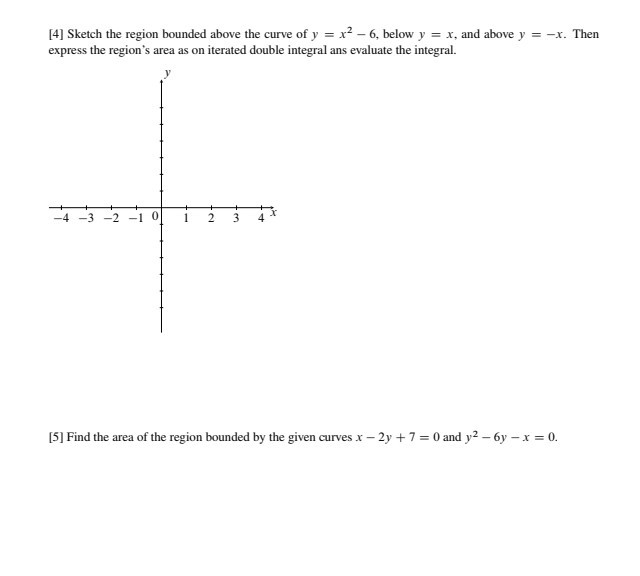Sketch the region bounded above the curve of y = x2 - 6, below y = x, and above y = -x. Then express the region's area as on iterated double integral ans evaluate the integral. -4 -3 -2 -1 0 1 2 3 4  Find the area of the region bounded by the given curves x - 2y + 7 = 0 and y2 -6y - x = 0.

• ### 6. (4 pts) Consider the double integral∫R(x2+y)dA=∫10∫y−y(x2+y)dxdy+∫√21∫√2−y2−√2−y2(x2+y)dxdy.(a) Sketch the region of integration R in Figure 3.(b)...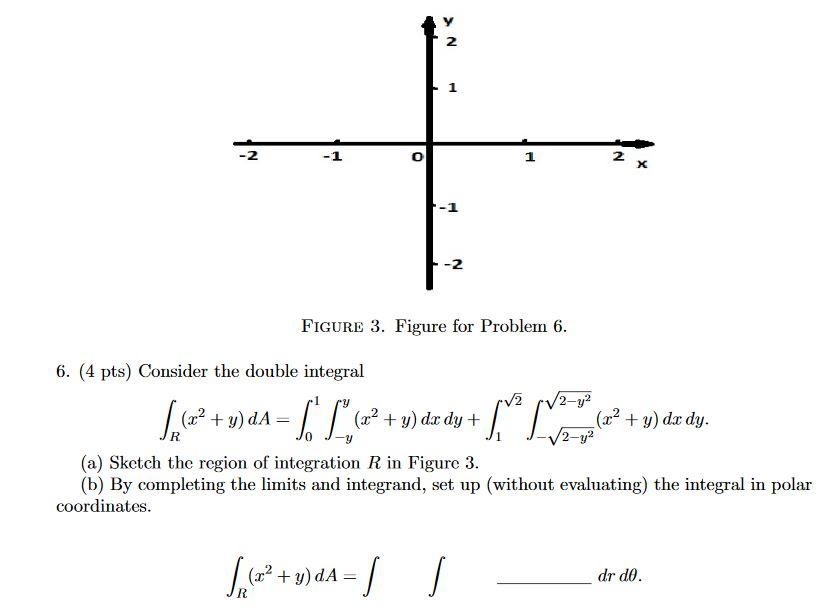6. (4 pts) Consider the double integral∫R(x2+y)dA=∫10∫y−y(x2+y)dxdy+∫√21∫√2−y2−√2−y2(x2+y)dxdy.(a) Sketch the region of integration R in Figure 3.(b) By completing the limits and integrand, set up (without evaluating) the integral in polar coordinates. 2 1 2 X -2 FIGURE 3. Figure for Problem 6. 6. (4 pts) Consider the double integral V2 2-y2 (2? + y) dA= (32 + y) dx dy + (x2 + y) dx dy. 2-y? (a) ketch the region of integration R in Figure 3. (b) By completing...

• ### please answer all of them thanks! Exercise 8.4 Let R be the region bounded by y...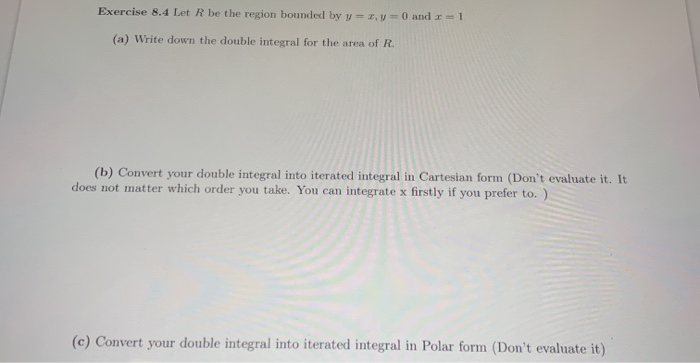please answer all of them thanks! Exercise 8.4 Let R be the region bounded by y = x, y = 0 and = 1 (a) Write down the double integral for the area of R. (b) Convert your double integral into iterated integral in Cartesian form (Don't evaluate it. It does not matter which order you take. You can integrate x firstly if you prefer to. ) (c) Convert your double integral into iterated integral in Polar form (Don't evaluate...